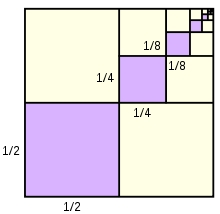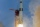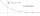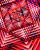# Sum 1-6

Find the sum of the geometric progression 3, 15, 75,… to six terms.

s6 =  11718

### Step-by-step explanation:Did you find an error or inaccuracy? Feel free to write us. Thank you!## Related math problems and questions:

• GP - three membersThe second and third of a geometric progression are 24 and 12(c+1), respectively, given that the sum of the first three terms of progression is 76. determine the value of c.
• Geometric progressiobIf the sum of four consective terms of geometric progression is 80 and arithmetic mean of second and fourth term is 30 then find terms?
• Arithmetic progression 2The 3rd term of an Arithmetic progression is ten more than the first term, while the fifth term is 15 more than the second term. Find the sum of the 8th and 15th terms of the Arithmetic progression if the 7th term is seven times the first term.
• Common differenceThe 4th term of an arithmetic progression is 6. If the sum of the 8th and 9th terms is -72, find the common difference.
• Missing term 2What is the missing term for the Geometric Progression (GP) 3, 15, 75,__, 1875?
• Find the 21Find the sum of the six terms of the finite geometric sequence 96, -48, 24, -12
• Sum of GP membersDetermine the sum of the GP 30, 6, 1.2, to 5 terms. What is the sum of all terms (to infinity)?
• Find the sumFind the sum of all natural numbers from 1 and 100, which are divisible by 2 or 5
• Three members GPThe sum of three numbers in GP (geometric progression) is 21 and the sum of their squares is 189. Find the numbers.
• GP - sequenceThe first three terms of a geometric sequence are as follows 10, 30. 90, find the next two terms of this sequence.
• Geometric progressionIn geometric progression, a1 = 7, q = 5. Find the condition for n to sum first n members is sn≤217.
• Six termsFind the first six terms of the sequence a1 = -3, an = 2 * an-1
• Sum of odd numbersFind the sum of all odd integers from 13 to 781.
• Sum of moneyOn a certain sum of money, invested at the rate of 10% compounded annually, the difference between the interests of the first year and the third year is 315 . Find the sum.
• Sum of the seventeen numbersThe sum of the 17 different natural numbers is 154. Determine the sum of the two largest ones.
• The sumThe sum of the first 10 members of the arithmetic sequence is 120. What will be the sum if the difference is reduced by 2?
• The sumThe sum of five consecutive odd numbers is 75. Find out the sum of the second and fourth of them.